# Difference between revisions of "Ethane"

  200 lightgrey Ethane.pdb  Ethane

Ethane.

## Models

The NERD parameters are:

 Molecule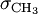$\sigma_{\mathrm {CH}_3}$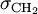$\sigma_{\mathrm {CH}_2}$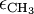$\epsilon_{\mathrm {CH}_3}$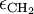$\epsilon_{\mathrm {CH}_2}$ ethane 3.825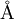$\mathrm{\AA}$ 3.93$\mathrm{\AA}$ 100.6 K

## Critical properties

The pressure, temperature and density at the critical point have been calculated for a virial equation of state using the TraPPE-UA force field, and are given in Table I of .

## Virial coefficients

The virial coefficients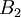$B_2$-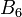$B_6$ as a function of temperature for the TraPPE-UA force field have been tabulated by Schultz and Kofke .

## Surface tension

The surface tension has been calculated for the TraPPE and OPLS force fields .

## Liquid-vapour equilibria

The Liquid-vapour equilibria has been calculated for the TraPPE and OPLS force fields .